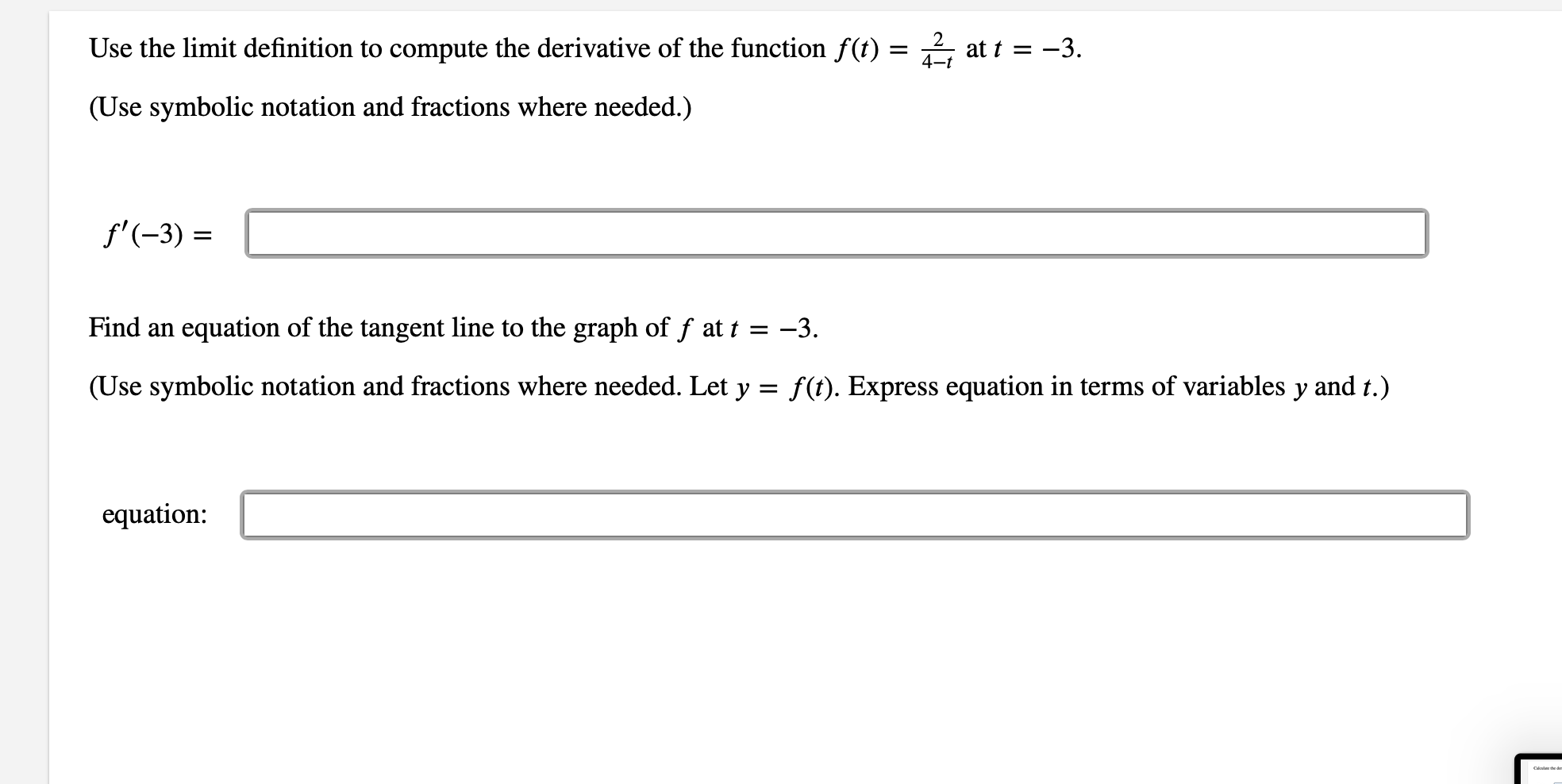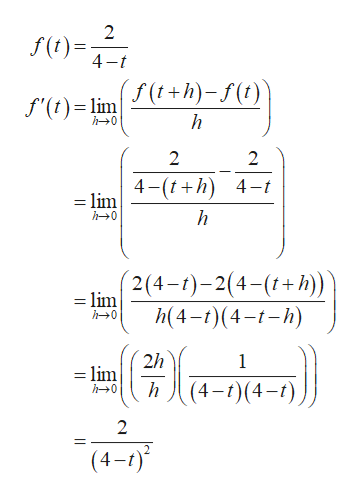# Use the limit definition to compute the derivative of the function f(t) = ^at t = -34-t(Use symbolic notation and fractions where needed.)f'(-3)Find anequation of the tangent line to the graph of f at t = -3.(Use symbolic notation and fractions where needed. Let y = f(t). Express equation in terms of variables y and t.)equation:

Question
72 viewshelp_outlineImage TranscriptioncloseUse the limit definition to compute the derivative of the function f(t) = ^ at t = -3 4-t (Use symbolic notation and fractions where needed.) f'(-3) Find an equation of the tangent line to the graph of f at t = -3. (Use symbolic notation and fractions where needed. Let y = f(t). Express equation in terms of variables y and t.) equation: fullscreen
check_circle

Step 1

The derivative of the functi...help_outlineImage Transcriptionclose2 f() 4- = f'(t)lim +h)-f(i) h h0 2 2 4-(th) 4- =lim h0 2(4-)-2(4-() = lim h(4-(4 ) h0 2h = lim h 1 (4-)(4-) h0 2 (4-) fullscreen

### Want to see the full answer?

See Solution

#### Want to see this answer and more?

Solutions are written by subject experts who are available 24/7. Questions are typically answered within 1 hour.*

See Solution
*Response times may vary by subject and question.
Tagged in

### Derivative